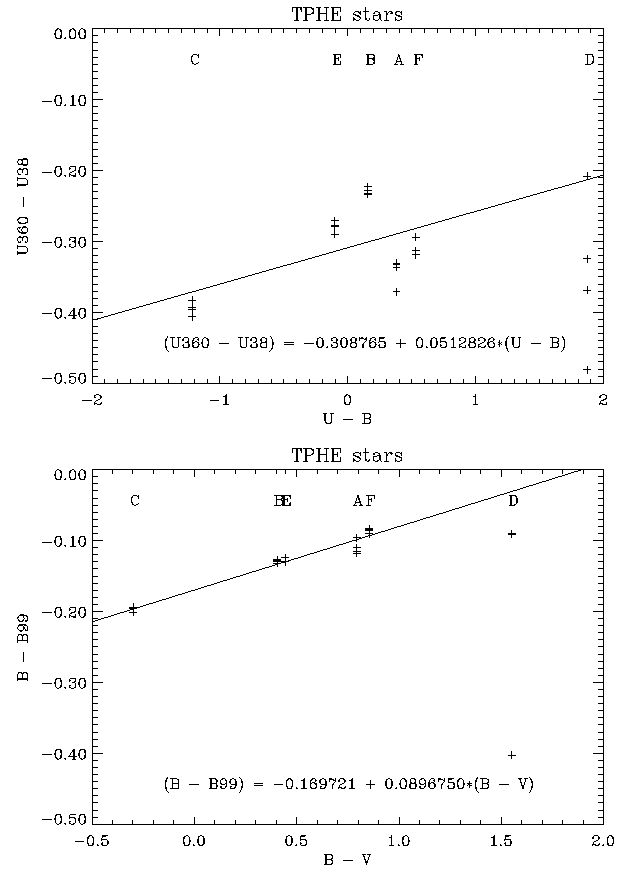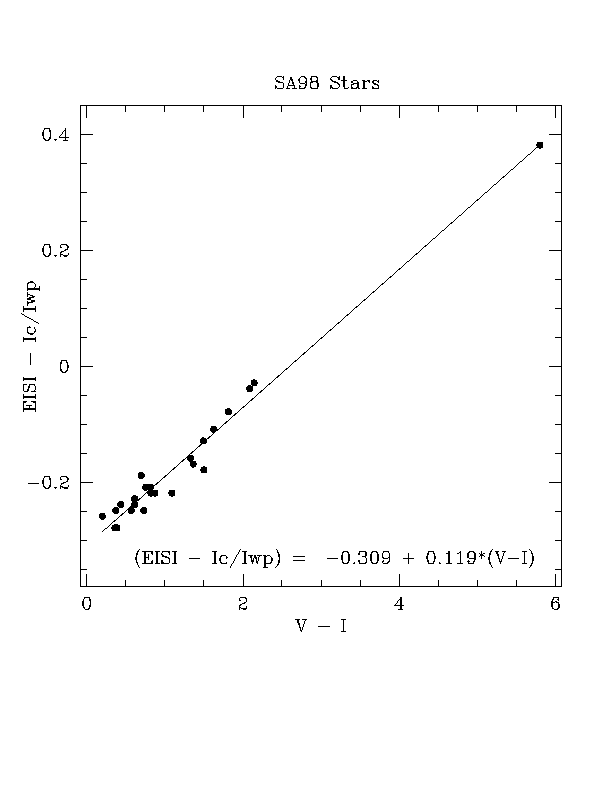# Conversion between standard U, B, V, R, I filters and WFI filters

To obtain the following equations the WFI magnitudes were corrected to extra-atmospheric values using atmospheric extinction coeffcicients obtained during the observing night. The frames were bias subtracted and flatfielded with twilight flats. The WFI magnitudes were calculated in ADU and not in electrons. Counting electrons would change the zero points by +0.75.

Notice the large color terms for the B filters. Such large terms will result in the impossibility of transforming to the standard system in some cases: for example, the zero point of a highly reddened star can differ by up to 0.15 magnitudes from the zero point of an unreddened star of the same color. Most Landolt standards have very moderate reddenings.

The transformation equations for the U filter have been simulated using Kurucz ATLAS9 model atmospheres and shown to be many-valued: the zero point depends on the luminosity class of the star varying by approximately 0.05 magnitudes going from a luminosity class V to a luminosity class I star. The difference could be as large as 0.1 mags for A-type stars.

U - U/38 = 21.65 + 0.12 x (U - B)

U - U/50 = 21.96 + 0.07 x (U - B)

B - B/99 = 24.53 + 0.31 x (B - V)

B - B/123 = 24.70 + 0.22 x (B - V)

V - V/89 = 24.12 - 0.09 x (B - V)

R - Rc/162 = 24.43 + 0.02 x (V - R)

I - Ic/Iwp = 23.06 + 0.18 x (V - I)

I - I/203 = 23.37 + 0.06 x (V - I)

 New Filters: U/50 and B/123

### Note: B/NEW == B/123, U/360 == U/50

U/50 - U/38 = -0.31 + 0.05 x (U - B) -> U - U/50 = 21.96 + 0.07 x (U - B)

B/123 - B/99 = -0.17 + 0.09 x (B - V) -> B - B/123 = 24.70 + 0.22 x (B - V)New Filters: I/203

### Note: EISI == I/203

I/203 - Ic/Iwp = -0.309 + 0.119 x (V - I) -> I - I/203 = 23.37 + 0.06 x (V - I)